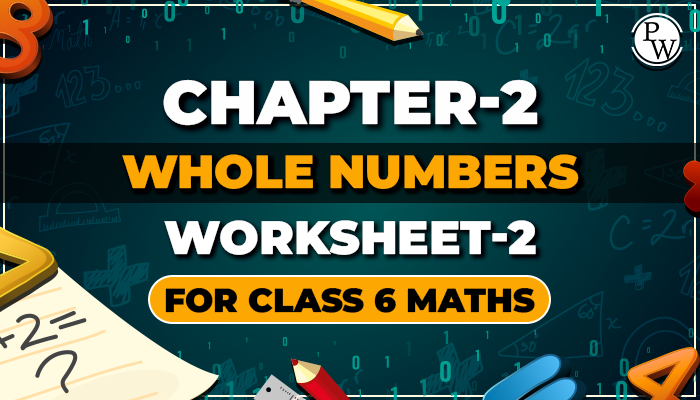# chapter-2 Whole Numbers worksheet-2 class 6 maths

#### Worksheets for Class 6 Maths

Whole numbers are a set of natural numbers that include zero. 0 is the smallest number. Whole numbers are 0, 1, 2, 3, ……… Natural numbers are whole numbers, but not all whole numbers are natural numbers.## Solved Examples

Q1. The numbers which we use for counting are known as.

1. Natural numbers
2. Prime numbers
3. Composite numbers
4. Rational numbers

Ans. Correct option is 1

The numbers 1, 2, 3, which we use for counting are known as natural numbers.

Q2. The product of a whole number and zero is?

1. Natural number
2. Whole number
3. Composite number
4. Whole number, natural number

Ans. Correct option is 2

If x is whole number

Then, x x 0=0

Q3. The sum of two natural numbers is?

1. Natural number
2. Whole number and natural number
3. Whole number
4. Irrational number

Ans. Correct option is 3

Since 1+2=3 ,1+1=2,2+2=4

3, 2, 4 are whole number and natural number.

Q4. The whole number between 0 to 5 are ?

1. 1,2,3,5
2. 0,1,2,3,4,5
3. 1,2,3,4
4. 1,3,5

Ans. Correct option is 3

ie., 1,2,3,4

Q5. The number with which when 10 is multiplied product remains the same

1. 0
2. -1
3. 1
4. 2

Ans. Correct option is 3

Since, 10 x 1 = 10

Q6. The multiplicative identity element of 20 is

1. 1
2. 0
3. -1
4. 2

Ans. Correct option is 1

Since, n x 1 = n

Q7. 2 x 3 = 3 x 2 is an example of

1. Closure property
2. Commutative property
3. Associative property
4. Distributive property

Ans. Correct option is 1

Since a x b = b x a is a general form of commutative property

Q8. Product of two even numbers is

1. Odd
2. Even
3. Both
4. None of them

Ans. Correct option is 2

Since 2 x 2 = 4 is an even number

Q9. The quotient of 25 /1 is

1. 1
2. 25
3. 24
4. 26

Ans. Correct option is 2

if a is any whole number ,then a/1 = a

Here 25 is a whole number.

Q10. 4 x (5-3) = (4 x 5) - (4 x 3) is an example of

1. Associative property
2. Commutative property
3. Distributive property of multiplication over addition
4. Distributive property of multiplication over subtraction

Ans. Correct option is 4

Since a x (b - c)=(a x b) - (a x c) is a general form of distributive property of mulplication over subtraction

Download worksheet-2 of class 9 maths chapter Whole Numbers and start solving the questions for reference use the solutions . Before solving the worksheet of Physics Wallah it is highly recommend reading the theory of chapter and solve the questions given in your textbook. Do follow NCERT Solutions for class 6 Maths prepared by expert faculty of Physics Wallah.

Students can also access the class 6 math notes from here. If any students need to take the online test to check their concepts or understanding then they can visit Quiz for Whole numbers.

Below link consist of class 6 Maths Chapter - 2 Whole Numbers download your free pdf copy from the below mentioned link of chapter Whole Numbers.